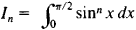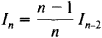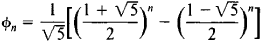# Recurrence Formula

The following article is from The Great Soviet Encyclopedia (1979). It might be outdated or ideologically biased.

## Recurrence Formula

(or recursion formula), a formula that reduces the computation of the nth term of some sequence (most often, a numerical sequence) to the computation of one or more of the terms preceding the nth term. The relevant preceding terms are usually found “near” the nth term, the number of the relevant preceding terms is independent of n, and the nth term can be expressed by means of these terms in a relatively simple manner. Recurrence formulas of a more complex nature, however, are possible. The general problems involved in recursion computations are the subject of the theory of recursive functions.

Examples. (1) The sequence Φn of Fibonacci numbers is defined by the formulas

Φ0 = 0

Φ1 = 1

Φπ+2 = Φπ+1 + Φπn ≥ 0

The last of these formulas is a recurrence formula and can be used to compute Φ2, Φ3, and subsequent terms of the sequence.

(2) SupposeIt can be easily proved that if n ≥ 2,This recurrence formula reduces the calculation of In to the calculation of I0 or I1 depending on whether η is odd or even.

Recurrence formulas usually provide a convenient computational scheme for finding the terms of a sequence one after another. Sometimes, however, an attempt is made to obtain on the basis of recurrence formula an “explicit” expression for the nth term of the sequence described by the formula. Thus, in the case of the Fibonacci numbers,References in periodicals archive ?
Thus, solutions exist as Frobenius-Fuchs series [5, 6] with recurrence formula for the coefficients reducing to two terms only in the case of the generalized Bessel differential equations.
And a practical method is proposed to determine the minimum embedding dimension and get the recurrence formula, with which a capacity model is built.
The recurrence formula for obtaining the sum at the next point is obtained by shifting the exponentials with 1 bin, subtracting the lower end and adding the upper end:
The following theorem gives a recurrence formula for the domination polynomial of (k, n)-path graphs.
Once the students discover the recurrence formula for the series coefficients, it's requested to build a Classpad300 program to automatically generate terms of the series.
A modification of the recurrence formula for the numbers [mathematical expression not reproducible] ([lambda]) and algorithms.
Let y([t.sub.1]) = 1; by recurrence formula (26), we can obtain all values of y([t.sub.i]); we calculate it in Table 1 for q = 3 and [N.sub.0] = 10.
(i) For w(x) = [(1 - x).sup.[alpha]][(1 + x).sup.[beta]], by using Fasenmyer's technique, the recurrence formula for the evaluation of the modified moments
Theorem 2 The (q, r)-Eulerian polynomials satisfy the following recurrence formula:
Using the obvious fact that for 1 < n < m and a, d [member of] Z with d [not equal to] 0, {a, a + d, ..., a + (n - 1) d} is a (2a+(n - 1) d)-symmetric set of the ring Z of integers, as a consequence of Theorem 2 we immediately obtain the following recurrence formula for the sums of powers on a finite arithmetic progression in Z.
Therefore, for n [greater than or equal to] 0, we obtain the recurrence formula in the form

Site: Follow: Share:
Open / Close